# A univariate polynomial class for R

## Introduction and summary

The following started as a straightforward programming exercise in operator overloading, but seems to be more generally useful. The goal is to write a polynomial class, that is a suite of facilities that allow operations on polynomials: addition, subtraction, multiplication, “division”, remaindering, printing, plotting, and so forth, to be conducted using the same operators and functions, and hence with the same ease, as ordinary arithmetic, plotting, printing, and so on.

The class is limited to univariate polynomials, and so they may therefore be uniquely defined by their numeric coefficient vector. The coefficients as a numeric vector can be extracted using the coef method for class "polynom", that is, using coef(p).

For simplicity the package is limited to real polynomials; handling polynomials with complex coefficients would be a simple extension. Dealing with polynomials with polynomial coefficients, and hence multivariate polynomials, would be feasible, though a major undertaking and the result would be very slow and of rather limited usefulness and efficiency.

### Relation to the previous package, polynom

The present package is intended to replace an earlier package called simply polynom, which provided similar functionality. The present package cannot be entirely a drop-in replacement, however, as it uses a function representation of polynomial objects rather than one that centres on the coefficient vector itself. The big advantage of the new representation is that polynomial objects may simply be used as R functions rather than requiring explicit coercion to function, as was needed in package polynom. In most other respects, however, the two packages behave similarly. (But note that it is not possible to use both packages simultaneously.)

New users should use the present package, PolynomF, for any new work or packages requiring polynomial operations. Package writers who made use of the polynom package, either as an import or as a dependency, are encourage to migrate their work to use PolynomF instead. Adaptation should be very straightforward. Moreover any future developments will occur in PolynomF; the previous package should now be considered legacy code.

# General orientation

The function polynomial(), (or alternatively, polynom()), creates an object of class "polynom" from a numeric coefficient vector. Coefficient vectors are assumed to apply to the powers of the carrier variable in increasing order, that is, in the truncated power series form, and in the same form as required by polyroot(), the system function for computing zeros of polynomials.1

Polynomials may also be created by specifying a set of $$(x, y)$$ pairs and constructing the Lagrange interpolation polynomial that passes through them (poly_calc(x, y)). If y is a matrix, an interpolation polynomial is calculated for each column and the result is a list of polynomials (of class polylist).

The third way polynomials are commonly generated is via its zeros using poly_calc(z), which creates the monic polynomial of lowest degree with the values in z as its zeros.

The core facility provided is the group method function Ops.polynom(), which allows arithmetic operations to be performed on polynomial arguments using ordinary arithmetic operators.

### Changes in versions 2.0-0 and 2.0-1

In this release, functions in previous releases using a period, "." in their name which were not S3 methods have been replaced by names using an underscore in place of the period. Thus the previously named function poly.calc has become poly_calc. The old names have been removed and now defunct.

One possibly useful addition has been the function poly_orth_general which allows sets of orthogonal polynomials to be generated using an inner product definition using an R function. This must be a function where the first two arguments are polynomial objects with the second having a default value equal to the first. Hence if the inner product function is called with just one polynomial argument it returns the squared $$L_2-$$norm.

Weighted discrete orthogonal polynomials can be included as a special case, and the inner product definition for this case serves as a template, as in this example, a special case of the Poisson-Charlier polynomials:

Discrete <- function(p, q = p, x, w = function(x, ...) 1, ...) sum(w(x, ...)*p(x)*q(x))
PC <- poly_orth_general(inner_product = Discrete, degree = 4,
x = 0:100, w = dpois, lambda = 1)
plot(PC, lwd = 2, legend = TRUE)
title(main = "Poisson-Charlier(1) polynomials to degree 4", font.main = 3)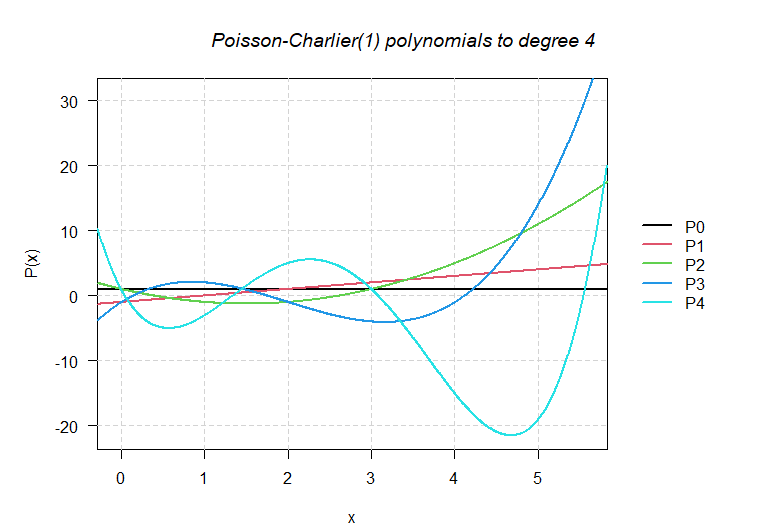This inner product function, along with some others for the classical orthogonal polynomials of mathematical physics are included, although if formulae for the recurrence relation are known, using them directly would be more efficient.

# Examples

## Miscellaneous computations using polynomial arithmetic

(p1 <- poly_calc(1:6))       ## a monic polynomial with given zeros
720 - 1764*x + 1624*x^2 - 735*x^3 + 175*x^4 - 21*x^5 + x^6
solve(p1)                    ## check that the zeros are as specified
 1 2 3 4 5 6
polyroot(coef(p1))           ## check using the Traub-Jenkins algorithm
 1-0i 2+0i 3+0i 4-0i 5+0i 6-0i
p2 <- -change_origin(p1, 3)  ## a negative shifted version of p1
plot(p1, xlim = c(-2.5, 6.5), ylim = c(-20, 20), lwd = 2, col = "steel blue", bty = "n")
lines(p2, col = "brown", lwd = 2)
abline(h = 0)
points(cbind(solve(p1), 0), pch = 1,  cex = 1,   col = "brown")
points(cbind(solve(p2), 0), pch = 19, cex = 0.5, col = "steel blue")
## stationary points
stat <- solve(deriv(p1))
lines(tangent(p1, stat), limits = cbind(stat-0.5, stat + 0.5),
lty = "solid", col = "black")
points(stat, p1(stat), pch = 19, cex = 0.5, col = "red")
stat <- solve(deriv(p2))
lines(tangent(p2, stat), limits = cbind(stat-0.5, stat + 0.5),
lty = "solid", col = "cadet blue")
points(stat, p2(stat), pch = 19, cex = 0.5, col = "red")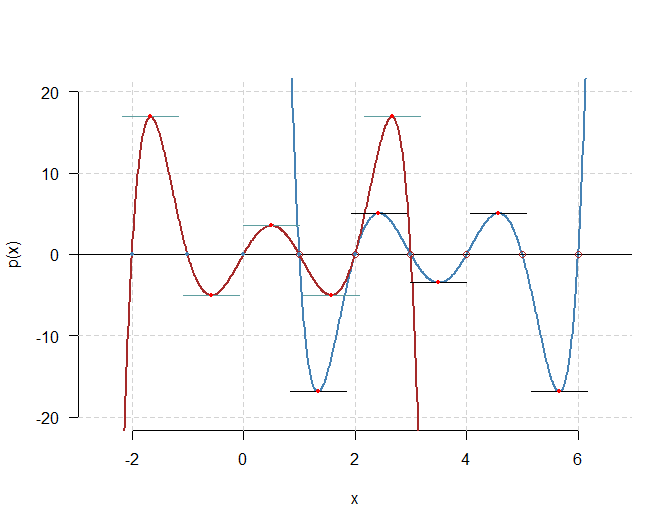## various checks
z <- (-2):6
setNames(p1(z), paste0("z=", z))
z=-2  z=-1   z=0   z=1   z=2   z=3   z=4   z=5   z=6
20160  5040   720     0     0     0     0     0     0
setNames(p2(z), paste0("z=", z))
z=-2   z=-1    z=0    z=1    z=2    z=3    z=4    z=5    z=6
0      0      0      0      0      0   -720  -5040 -20160
setNames((p1*p2)(z), paste0("z=", z))
z=-2 z=-1  z=0  z=1  z=2  z=3  z=4  z=5  z=6
0    0    0    0    0    0    0    0    0
p3 <- (p1 - 2 * p2)^2                         ## moderately complicated expression.
setNames(p3(0:4), paste0("z=", 0:4))          ## should have zeros at 1, 2, 3
z=0     z=1     z=2     z=3     z=4
518400       0       0       0 2073600 

## Discrete orthogonal polynomials

Find the orthogonal polynomials on $$x_0 = (0, 1, 2, 3, 5)$$ and construct R functions to evaluate them at arbitrary $$x$$ values.

x0 <- c(0:3, 5)
op <- poly_orth(x0, norm = TRUE)
plot(op, lwd = 2, legend = TRUE)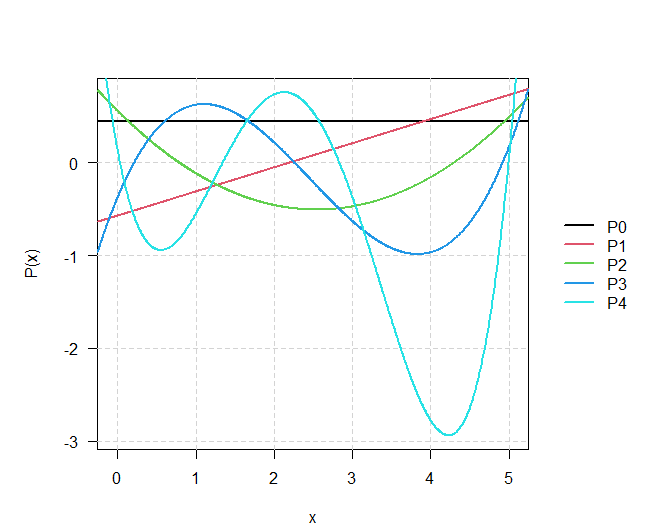fop <- as.function(op)        ## Explicit coercion needed for polylist
zap(crossprod(fop(x0)))       ## Verify orthonormality
P0 P1 P2 P3 P4
P0  1  0  0  0  0
P1  0  1  0  0  0
P2  0  0  1  0  0
P3  0  0  0  1  0
P4  0  0  0  0  1

## Chebyshev polynomial approximation I

The Chebyshev polynomials of the first kind, $$T_n(x)$$, like all orthogonal sets, satisfy a 2-term recurrence relation: \begin{aligned} T_0(x) &= 1,\quad T_1(x) = x,\\ T_n(x) &= 2x T_{n-1}(x) - T_{n-2}(x), \qquad n = 2, 3, \ldots \end{aligned} The orthogonality relation is then: $\int_{-1}^1\frac1{\sqrt{1-x^2}}T_n(x)T_m(x)dx = c_n\delta_{nm}$ where $$\delta_{nm}$$ is the usual Kronecker delta function, and $$c_0 = \pi, c_n = \pi/2, n = 1, 2, \ldots$$. To approximate a function, $$f(x)$$ on the range $$-1 < x < 1$$, the Chebyshev approximation of the first kind uses a polynomial of the form $f(x) \approx \sum_{j=0}^N \beta_jT_j(x)$ where the coefficients are given by $\beta_j = \int_{-1}^1\frac1{\sqrt{1-x^2}}T_j(x)f(x)dx / c_j$ We now explore the quality of this approximation for the central part of a $$N(0,\sigma=\frac14)$$ density using $$N=14$$, (possibly a little overkill!).

x <- polynomial()
Tr <- polylist(1, x)
for(j in 3:15) {
Tr[[j]] <- 2*x*Tr[[j-1]] - Tr[[j-2]]
}
Tr <- setNames(Tr, paste0("T", sub(" ", "_", format(seq_along(Tr)-1))))

The necessary inner product function has been supplied as part of the package, slightly indirectly, but are easy to specify anyway

ChebyT <- function(p, q = p) {
integrate(function(x) 1/sqrt(1-x^2)*p(x)*q(x), lower = -1, upper = 1,
subdivisions = 500, rel.tol = .Machine$double.eps^0.5)$value
}
zap(outer(Tr, Tr, Vectorize(ChebyT))*2/pi) ## check of orthogonality
T_0 T_1 T_2 T_3 T_4 T_5 T_6 T_7 T_8 T_9 T10 T11 T12 T13 T14
T_0   2   0   0   0   0   0   0   0   0   0   0   0   0   0   0
T_1   0   1   0   0   0   0   0   0   0   0   0   0   0   0   0
T_2   0   0   1   0   0   0   0   0   0   0   0   0   0   0   0
T_3   0   0   0   1   0   0   0   0   0   0   0   0   0   0   0
T_4   0   0   0   0   1   0   0   0   0   0   0   0   0   0   0
T_5   0   0   0   0   0   1   0   0   0   0   0   0   0   0   0
T_6   0   0   0   0   0   0   1   0   0   0   0   0   0   0   0
T_7   0   0   0   0   0   0   0   1   0   0   0   0   0   0   0
T_8   0   0   0   0   0   0   0   0   1   0   0   0   0   0   0
T_9   0   0   0   0   0   0   0   0   0   1   0   0   0   0   0
T10   0   0   0   0   0   0   0   0   0   0   1   0   0   0   0
T11   0   0   0   0   0   0   0   0   0   0   0   1   0   0   0
T12   0   0   0   0   0   0   0   0   0   0   0   0   1   0   0
T13   0   0   0   0   0   0   0   0   0   0   0   0   0   1   0
T14   0   0   0   0   0   0   0   0   0   0   0   0   0   0   1

The approximating polynomial for the normal density can now be found and tested.

fx <- function(x) dnorm(x, sd = 0.25)
b <- sapply(Tr, ChebyT, q = fx)/sapply(Tr, ChebyT)
fx_approx <- sum(b * Tr)
curve(fx, xlim = c(-1,1))
lines(fx_approx, col = "red", limits = c(-1, 1))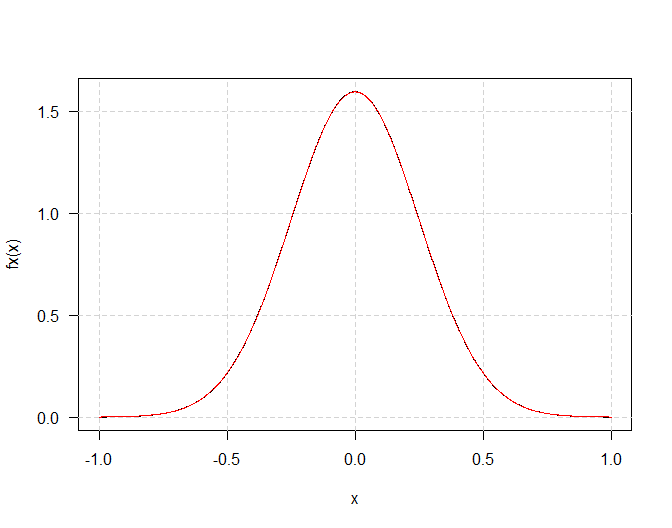curve(1e4*(fx(x) - fx_approx(x)), xlim = c(-1,1)) ## error pattern x 10000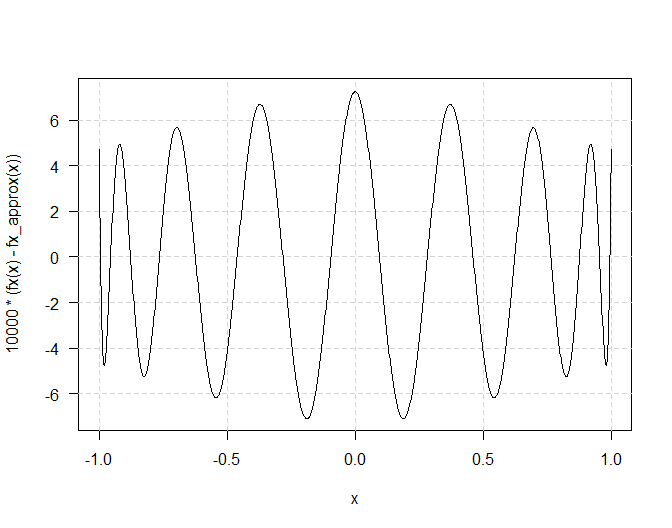The approximation may be extended to the cumulative distribution function:

Fx <- function(x) pnorm(x, sd = 0.25)
Fx_approx <- integral(fx_approx) + 0.5
curve(Fx, xlim = c(-1, 1))
lines(Fx_approx, col = "red", limits = c(-1,1))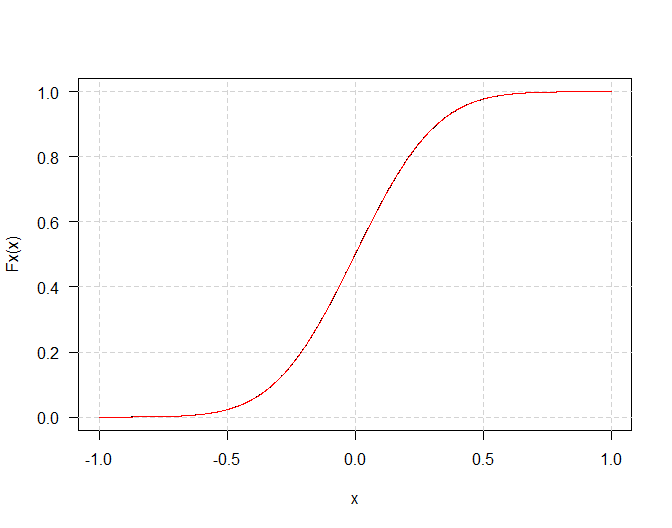curve(1e4*(Fx(x) - Fx_approx(x)), xlim = c(-1,1)) ## error pattern x 10000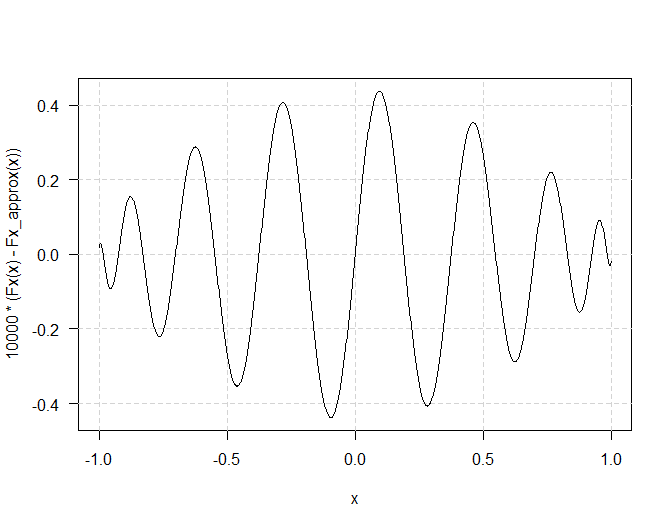## Chebyshev polynomial approximation II

Functions that “bend the other way”, Chebyshev polynomials of the second kind can sometimes be more useful. These have the same recurrence relation as those of the first kind, but a different starting set and a different, but similar looking orthogonality relationship. \begin{aligned} U_0(x) &= 1,\quad U_1(x) = 2x,\\ U_n(x) &= 2x U_{n-1}(x) - U_{n-2}(x), \qquad n = 2, 3, \ldots\\ \int_{-1}^1&\sqrt{1-x^2}U_n(x)U_m(x)dx = \frac{\pi}2\delta_{nm} \end{aligned} We use these polynomials to build a polynomial approximation to the arc sine function, $$f(x) = \sin^{-1}x$$. The process is entirely analogous to the one outlined previously.

x <- polynomial()
Ur <- polylist(1, 2*x)

for(j in 3:15) {
Ur[[j]] <- 2*x*Ur[[j-1]] - Ur[[j-2]]
}
Ur <- setNames(Ur, paste0("U", sub(" ", "_", format(seq_along(Ur)-1))))
plot(Ur, ylim = c(-2,2))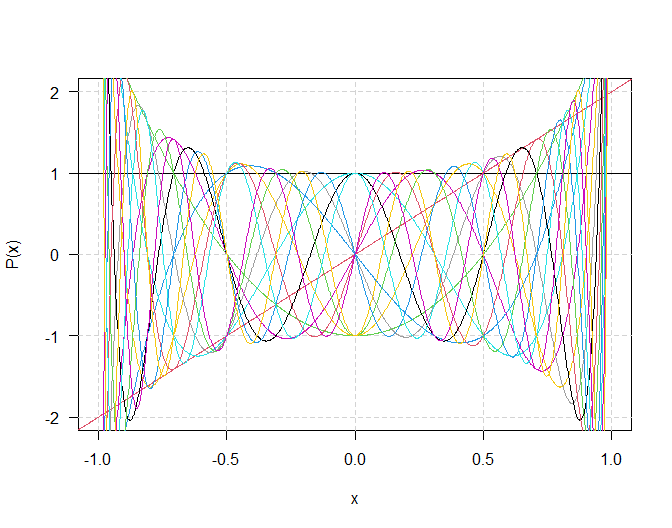ChebyU <- function(p, q = p) {
integrate(function(x) sqrt(1-x^2)*p(x)*q(x), lower = -1, upper = 1,
subdivisions = 500, rel.tol = .Machine$double.eps^0.5)$value
}
b <- sapply(Ur, ChebyU, q = asin)/(pi/2)
asin_approx <- sum(b * Ur)

curve(asin, xlim = c(-1,1))
lines(asin_approx, col = "red", limits = c(-1,1))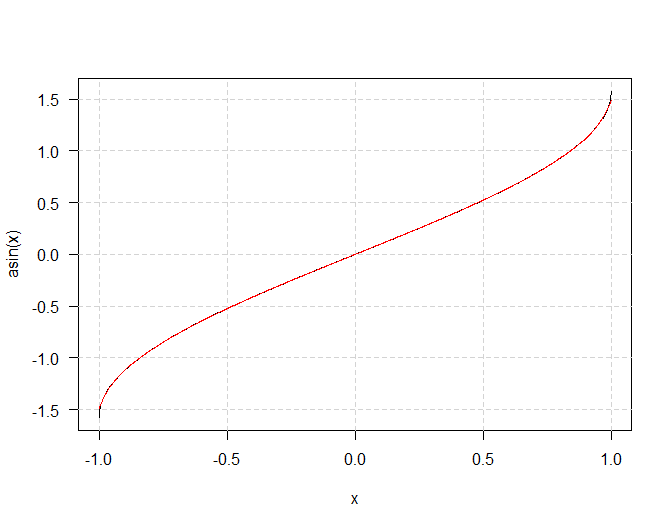curve(1e4*(asin(x) - asin_approx(x)), xlim = c(-1,1), ylim = c(-50,50)) ## errors by 10000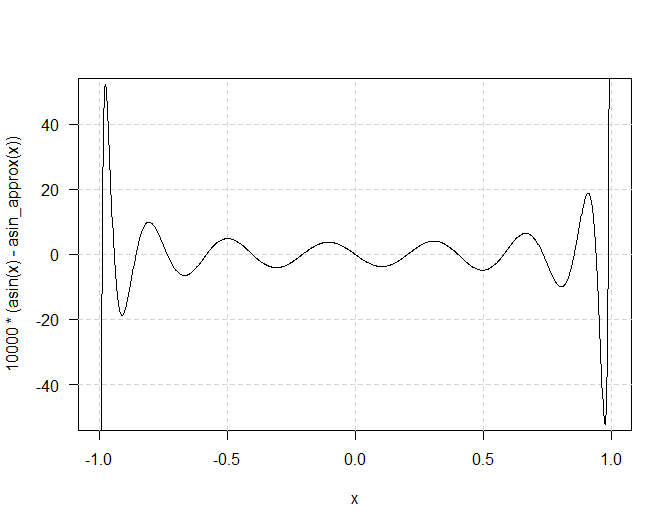Overall the approximation is not especially good, and degrades considerably close to the ends of the range where the derivative of the function itself becomes unbounded.

## A simple branching process

If an organism can have $$0, 1, 2, ...$$ offspring with probabilities $$p_0 , p_1 , p_2 , ...$$ the generating function for this discrete distribution is defined as $$P ( s ) = p_0 + p_1 s + p_2 s^2 + \cdots$$. If only a (known) finite number of offspring is possible, the generating function is a polynomial. In any case, if all offspring themselves reproduce independently according to the same offspring distribution, then the generating function for the size of the second generation can be shown to be $$P ( P ( s ))$$, and so on. There is a nice collection of results connected with such simple branching processes: in particular the chance of ultimate extinction is the (unique) root between 0 and 1 of the equation $$P ( s )-s = 0$$. Such an equation clearly has one root at $$s = 1$$, which, if $$P ( s )$$ is a finite degree polynomial, may be “divided out”. Also the expected number of offspring for an organism is clearly the slope at $$s=1$$, that is, $$P'(1)$$.

Consider a simple branching process where each organism has at most three offspring with probabilities $\textstyle p_0 = {\textstyle \frac1{10}},\quad p_1 = {\textstyle \frac5{10}},\quad p_2 = {\textstyle \frac3{10}},\quad p_3={\textstyle \frac1{10}},\quad p_4 = p_5 = \cdots = 0.$ The following will explore some of its properties without further discussion.

P <- polynomial(c(1, 5, 3, 1))/10
s <- polynomial(c(0, 1))
(mean_offspring <- deriv(P)(1))
 1.4
pretty_poly <- bquote(italic(P)(italic(s)) == ' '*
.(parse(text = gsub("x", "italic(' '*s)", as.character(P)))[]))
plot(s, xlim = c(0,1), ylim = c(0,1), bty = "n", type = "n", main = pretty_poly,
xlab = expression(italic(s)), ylab = expression(italic(P)(italic(s))))
x <- c(0,1,1,0)
y <- c(0,0,1,1)
segments(x, y, 1-y, x, lty = "solid", lwd = 0.2)
lines(s, limits = 0:1, col = "grey")
lines(P, limits = 0:1)
lines(tangent(P, 1), col = "red", limits = c(0.5, 1.0), lwd = 1.5)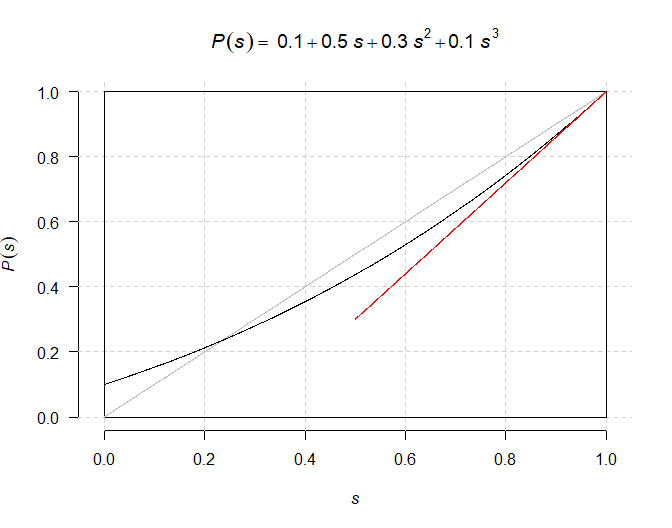(ep <- solve((P - s)/(1 - s))) ## extinction; factor our the known zero at s = 1
 -4.236068  0.236068
ex <- ep                 ## extract the appropriate value (may be complex, in general)
plot(s, xlim = c(0,1), ylim = c(0,1), type = "n", bty = "n", main = pretty_poly,
xlab = expression(italic(s)), ylab = expression(italic(P)(italic(s))))
segments(x, y, 1-y, x, lty = "solid", lwd = 0.2)
lines(s,     col = "grey", limits = 0:1)  ## higher generations
lines(P,          col = 1, limits = 0:1)
lines(P(P),       col = 2, limits = 0:1)
lines(P(P(P)),    col = 3, limits = 0:1)
lines(P(P(P(P))), col = 4, limits = 0:1)
arrows(ex, P(ex), ex, par("usr"), angle = 15, length = 0.125)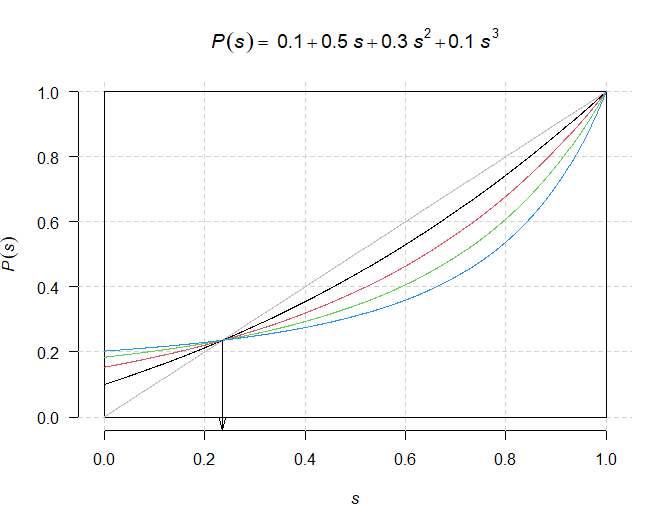## Polynomials can be numerically fragile

This can easily lead to surprising numerical problems.

x0 <- 80:89
y0 <- c(487, 370, 361, 313, 246, 234, 173, 128, 88, 83)
p <- poly_calc(x0, y0)        ## leads to catastropic numerical failure!
range(p(x0) - y0)             ## these should be "close to zero"!
 -2.5 96.5
p1 <- poly_calc(x0 - 84, y0)  ## changing origin fixes the problem
range(p1(x0 - 84) - y0)       ## these are 'close to zero'.
 -2.199840e-11  7.389644e-12
plot(p1, xlim = c(80, 89) - 84, xlab = "x0 - 84")
points(x0 - 84, y0, col = "red")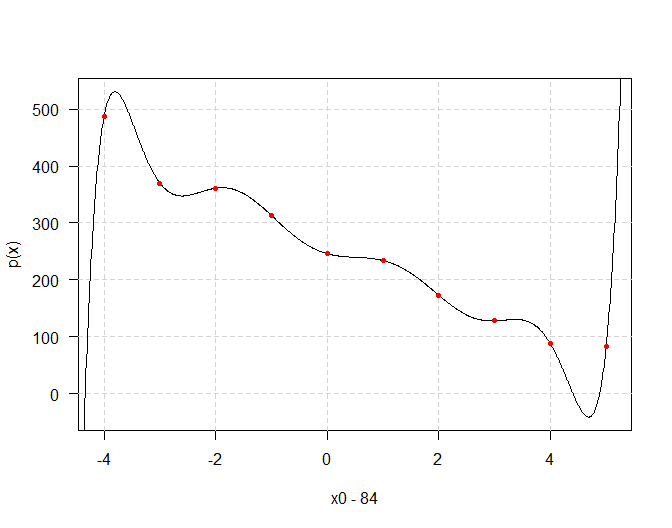## Can we now write the polynomial in "raw" form?
p0 <- change_origin(p1, -84)  ## attempting to change the origin back to zero
## leads to severe numerical problems again
plot(p0, xlim = c(80, 89))
points(x0, y0, col = "red")   ## major errors due to finite precision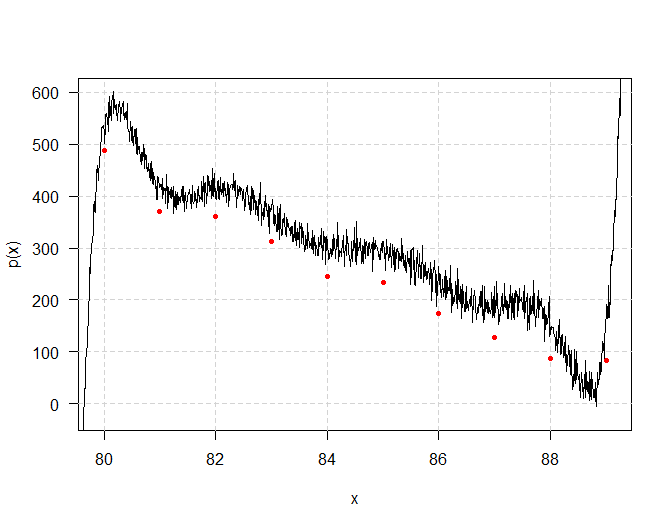1. As a matter or terminology, the zeros of the polynomial $$P(x)$$ are the same as the roots of equation $$P(x) = 0$$.↩︎